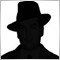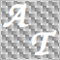• 概述
• 评论 (2)
• 评论 (2)

AvtoFiboLevelsDemo

The indicator is created for professional trading by Fibonacci levels. AutoFiboLevels plots Fibonacci retracement and extension levels on impulse and corrective wave. The indicator unambiguously interprets a market situation. Points of extremum for level plotting are calculated using fractal analysis. A trader can independently set retracement and extension levels and calculation time frame adjusting the indicator for various strategies.

This version of the indicator works only on EURUSD. Full version of the indicator.

Parameters

• WorkTF - time frame for indicator calculation, if (= 0) - current time frame;
• fiboPercLevel_1...11 - Fibonacci retracement levels;
• upFiboLevelColor - Color of upward grid lines of Fibonacci retracement levels;
• dnFiboLevelColor - Color of downward grid lines of Fibonacci retracement levels;
• ShowFiboImpuls - if (= true) - display Fibonacci retracement levels on an impulse wave;
• ShowFiboCorrection - if (= true) - display Fibonacci retracement levels on a corrective wave;
• FiboShowCorrect - level of an impulse level retracement, Fibonacci retracement levels are displayed on a corrective wave when this level is reached and if ShowFiboCorrection = true;
• ShowExpImpuls - if (= true) - display impulse wave extension level;
• ShowExpCorrection - if (= true) - display corrective wave extension level;
• fiboPercExpansion_1...5 - Fibonacci extensions;
• upFiboExpansionColor - Color of upward grid lines of Fibonacci extensions;
• dnFiboExpansionColor - Color of downward grid lines of Fibonacci extensions.12019
2017.11.25 20:3421304
2016.08.31 09:20